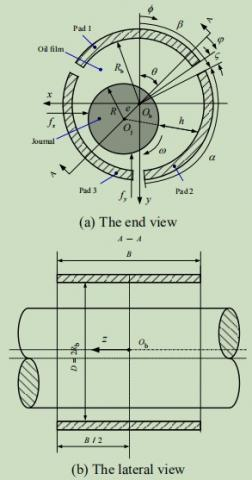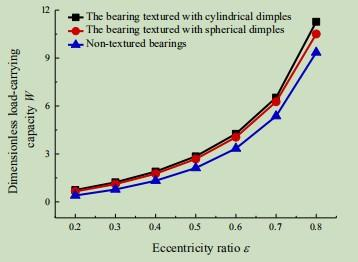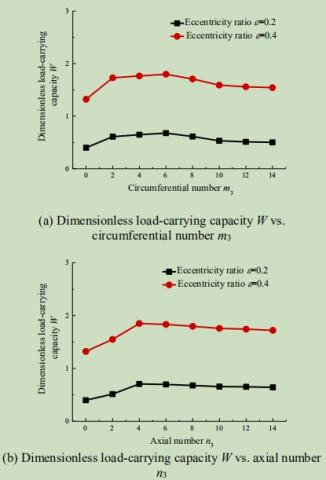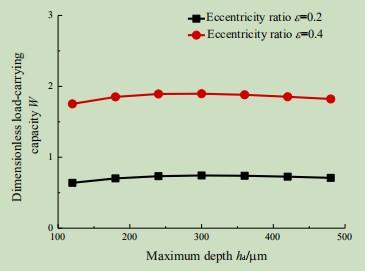# Optimal surface texture design of journal bearing with axial grooves

Optimal surface texture design of journal bearing with axial grooves

Bian Guo

School of Mechanical Engineering, Baoji University of Arts and Sciences, Baoji 721016, China

Corresponding Author Email:
gguo2006@126.com
Page:
267-272
|
DOI:
https://doi.org/10.18280/ijht.350206
|
Accepted:
|
Published:
30 June 2017
| Citation

OPEN ACCESS

Abstract:

This paper conducts a numerical investigation of the effects of micro spherical and cylindrical dimples on the load-carrying capacity of the journal bearing with axial grooves, aiming to obtain the best lubrication performance. In order to analyze the lubrication performance, the mass conservative JFO (Jakobsson, Floberg,Olsson) cavitation boundary condition is adopted to address the oil film rupture and reformulation, and the Reynolds equation is used to build the hydrodynamic lubrication model for the dimple-textured journal bearing with axial grooves. On this basis, the author examines how the shape, location and geometric parameters of the dimples influence the load-carrying capacity at various eccentricities. The results show that the dimples can significantly improve the load-carrying capacity of the bearing, especially when the dimples are textured on bearing pad 3, and that the maximum load-carrying capacity can be obtained with micro cylindrical dimples of area ratio s=0.5, maximum depth hd=300 μm, circumferential number m3= 6, and axial number n3= 4.

Keywords:

surface texture, journal bearing, jfo boundary condition, load-carrying capacity

1. Introduction

The journal bearing with axial grooves is extensively used in high-performance diesel engine, gas turbine shafting and fuel pump. In order to improve the performance and service life of the engines, more and more attention has been paid to improving the lubrication performance, a key determinant of engine performance, of the journal bearing with axial grooves [1-2]. With the development of surface etching and laser technique in recent years, the micro dimples have been applied to increase the load-carrying capacity of modern machine components thanks to its secondary lubrication and micro-hydrodynamic effects. It is widely recognized that texturing well-designed dimples on the surface of journal bearing is a promising way to improve the load-carrying capacity [3-8].

Since the lubrication performance hinges on the shape, location and geometric parameters of the dimples, domestic and foreign scholars have spared no effort in the search for the appropriate dimple shape, location and geometric parameters. Li Y. N. et al.  probed into the effect of the geometric parameters, density and location of rectangular surface texture on the tribological properties of journal bearing. Tala-Ighil N. et al.  analyzed the impact of spherical dimple location on the load-carrying capacity of journal bearing, suggesting that the dimples at the maximum film pressure could improve the load-carrying capacity and reduce the friction coefficient of the bearing. Li J. H. et al. discussed the influence of the width, depth and area rate of rectangular grooves on the load-carrying capacity and frictional resistance coefficient of journal bearing, and concluded that the optimal depth of grooves should be 25mm to achieve the maximum load-carrying capacity and minimum frictional resistance coefficient. Gao Y. et al.  obtained the optimal dimple shape, depth and location through the investigation on how the journal bearing lubricity is affected by the distribution pattern and dimensional parameters of rectangular grooves, rectangular concave pits and circular concave pits. Liu J. et al.  explored the effect of the trapezoidal herringbone grooves on the load-carrying capacity and the friction of journal bearing, and found that the number and trapezoidal angle of the grooves have a minimal impact on the load-carrying capacity, but a direct bearing on friction. Comparing the friction coefficients of radial journal bearings with transverse, circumferential and herringbone grooves, Adatepe H. et al.  disclosed that circumferential and herringbone grooves could effectively reduce the friction coefficient of the bearing. Ashihara K. et al.  studied the effect of groove depth on friction coefficient, oil film cooling effect and the flow rate of journal bearing, and arrived at the optimal depth of grooves. Khatri Chandra B. et al.  examined the influence of spherical pits over the lubricity and operation stability of journal bearing under combined pressure, pointing out that spherical pits could reduce the friction torque and damping coefficient and increase the stiffness coefficient of the bearing.

Focusing on the full circle journal bearing, the abovementioned studies mostly tackled oil film rupture issue with the Reynolds or Swift-Stieber boundary condition. In actual working conditions, however, the full circle journal bearing features poor stability at a high speed, and the oil film will form again after rupturing. In other words, the bearing has not only the rupture boundary but also the reformulation boundary during the operation. To overcome the poor high- speed stability of the full circle journal bearing, axial grooves are designed on the inner surface of the bearing to prevent pressure perturbation in the axial direction. Compared with the full circle journal bearing, the journal bearing with axial grooves has better stability but weaker load-carrying capacity. Targeted at the low load-carrying capacity of journal bearing with axial grooves, this paper textures micro dimples on the surface of bearing pads, adopts the mass conservative JFO boundary condition to address the oil film rupture and reformulation, and uses the Reynolds equation to build the hydrodynamic lubrication model for the dimple-textured journal bearing with axial grooves. Moreover, the effects of the dimple’s shape, location and geometric parameters on the load-carrying capacity are investigated to obtain the maximum load-carrying capacity. This research lays the theoretical basis for optimal surface texture design of journal bearing with axial grooves.

2. Theoretical Analysis

2.1 Geometric model

## 1.jpgFigure 1. Sketch map and coordinates of the journal bearing with three axial grooves

The journal bearing with three axial grooves is illustrated in Figure 1, where Ob and Oj stand for the bearing and journal center, respectively; f and φ refer to the oil film position from the negative direction of Y axis and offset line,respectively; θ and e denote the offset angle and eccentricity,respectively; Rb and R represent the radiuses of the bearing and the journal, respectively; ω means the angular speed of the journal; B and D signify the width and diameter of the bearing, respectively; plus, β is the initial position angle, ζ is the axial groove width angle, and α is the pad angle.

Since spherical and cylindrical pits are easy to process, the array of such pits is textured on the surface of each bearing pad. The bearing pad with spherical and cylindrical pits array is shown in Figure 2, where mi and ni denote the number of pits in the circumferential and axial directions, respectively;rd stands for the radius, hd refers to the maximum depth of pits;ξÕη represents the local-coordinate system in pit center. For the sake of simplicity, each pit is assumed to be located in a square unit with the length of lc. Hence, the area ratio of each pit can be expressed as:

$s=\frac{\pi r_{\mathrm{d}}^{2}}{l_{\mathrm{c}}^{2}}$     (1)

## 2.jpgFigure 2. Sketch map of bearing pad i textured with spherical and cylindrical dimples

2.2 Governing equations

The lubrication behavior of the oil film in the journal bearing with three axial grooves is analyzed by the Reynolds equation based on the laminar flow, constant temperature and the lubrication hypothesis of incompressible Newtonian fluid. The dimensional Reynolds equation of bearing pad i can be expressed as :

$\frac{\partial}{\partial x}\left(\frac{h_{i}^{3}}{\mu} \frac{\partial p_{i}}{\partial x}\right)+\frac{\partial}{\partial z}\left(\frac{h_{i}^{3}}{\mu} \frac{\partial p_{i}}{\partial z}\right)=6 \mu \frac{\partial h_{i}}{\partial x}$     (2)

where, pi is the oil film pressure of bearing pad i; μ is the oil film dynamic viscosity; hi is the oil film thickness, which is obtained by formula (3).

$h_{i}=\left\{\begin{array}{ll}{1+\varepsilon \cos \varphi} & {(x, z) \notin \Omega} \\ {1+\varepsilon \cos \varphi+h_{t i}} & {(x, z) \in \Omega}\end{array}\right.$         (3)

where, ε equals the quotient of e divided by c and denotes the eccentricity ratio; c is the radial clearance, Ω is the texture area; hti is the oil film thickness of the pit, and is given by formula (4).

$h_{t,i}=\left\{\begin{array}{lr}{\sqrt{\left(\frac{r_{\mathrm{d}}^{2}+h_{\mathrm{d}}^{2}}{2 h_{\mathrm{d}}}\right)^{2}-\left(\xi^{2}+\eta^{2}\right)}-\frac{r_{\mathrm{d}}^{2}-h_{\mathrm{d}}^{2}}{2 h_{\mathrm{d}}}} & {\text {spherical}} \\ {h_{\mathrm{d}}} & {\text {cylindrical}}\end{array}\right.$    (4)

According to the JFO theory, the oil film will break into strips when the oil film pressure falls below the cavitation pressure, and the lubricating area is divided into the full fluid film region and the cavitation region. The full fluid film region is covered only by the lubricative oil film. The lubrication behavior in this region is expressed by formula (2); the cavitation region, however, is filled up with oil and gas mixture, and the oil film pressure is equal to the constant cavitation pressure. The lubrication behavior in this region is governed by the equation below:

$6 \mu \frac{\partial h_{i}}{\partial x}=0$    (5)

In order to determine the rupture boundary and reformulation boundary of the oil film, the pressure distribution at the two boundaries must satisfy the Reynolds cavitation boundary condition and JFO conservation of mass boundary, respectively  :

$\left\{\begin{array}{l}{p_{i}=p_{c}} \\ {\frac{\partial p_{i}}{\partial n}=0}\end{array}\right.$    (6)

$\frac{h_{i}^{2}}{12 \mu} \frac{\partial p_{i}}{\partial n}=\frac{v_{n}}{2}\left(1-\frac{\rho}{\rho_{\mathrm{c}}}\right)$    (7)

where n is the normal vector of the oil film’s rupture boundary and reformulation boundary; vn is the oil film’s normal velocity; ρc is the oil film density in the cavitation region; ρ is the overall oil film density; pc is the cavitation pressure.For the convenience of analysis, the lubrication governing equations in the full fluid film region and the cavitation region should be nondimensionalized. For this purpose, the dimensionless variables are defined as follows:

$\varphi=x / R_{\mathrm{b}}, \quad \lambda=2 z / B, \quad H_{i}=\frac{h_{i}}{c}, \quad P_{i}=\frac{p \psi^{2}}{2 \omega \mu}$    (8)

where λ is the dimensionless axial coordinate; Pi is the dimensionless pressure; Hi denotes the dimensionless oil film thickness; ψ equals the quotient of c divided by Rb and denotes the clearance ratio. Substitute formula (8) into formula (2) and formula (5) to obtain the lubrication governing equations in the two regions:

$\frac{\partial}{\partial \varphi}\left(H_{i}^{3} \frac{\partial P_{i}}{\partial \varphi}\right)+\frac{\partial}{\partial \lambda}\left(H_{i}^{3} \frac{\partial P_{i}}{\partial \lambda}\right)=3 \frac{\partial H_{i}}{\partial \varphi}$    (9)

$3 \rho_{\mathrm{c}} \frac{\partial H_{i}}{\partial \varphi}=0$    (10)

During the solution of the dimensionless lubrication governing equations (9) and (10) in the two regions, the oil film’s rupture boundary and reformulation boundary are automatically identified by the JFO boundary condition and Payvar-Salant method. The function g and variable Fi are defined as follows :

$F_{i}=\left\{\begin{array}{ll}{\frac{\rho_{\mathrm{c}}}{\rho}-1} & {\text { (the cavitation region) }} \\ {\frac{P_{i}-P_{\mathrm{c}}}{P_{0}}} & {\text { (the full fluid film region) }}\end{array}\right.$    (11)

$g=\left\{\begin{array}{ll}{0} & {\left(F_{i}<0\right)} \\ {1} & {\left(F_{i} \geq 0\right)}\end{array}\right.$    (12)

where P0 is the dimensionless atmospheric pressure; Pc is the dimensionless cavitation pressure. The lubrication governing equations in the full fluid film region and the cavitation region can be combined into the following equation:

$\frac{\partial}{\partial \varphi}\left(P_{0} H_{i}^{3} \frac{\partial\left(F_{i} g\right)}{\partial \varphi}\right)+\left(\frac{D}{B}\right)^{2} \frac{\partial}{\partial \lambda}\left(P_{0} H_{i}^{3} \frac{\partial\left(F_{i} g\right)}{\partial \lambda}\right)=3\left[1+(1-g) F_{i}\right] \frac{\partial H_{i}}{\partial \varphi}$    (13)

Through numerical calculation, the boundary conditions of the film pressure distribution of bearing pad i are determined as follows:

$\left\{\begin{array}{l}{\left.P_{i}\right|_{\lambda=\pm 1}=0} \\ {\left.P_{i}\right|_{\varphi=\beta+(i-1) \alpha+i \zeta}=0} \\ {\left.P_{i}\right|_{\varphi=\beta+i \alpha+i \zeta}=0}\end{array}\right.$    (14)

The dimensionless pressure Pi of the bearing pad can be obtained by solving the unified lubrication governing equation (13) of bearing pad i in the full fluid film region and the cavitation region. Then, the dimensionless oil-film force Fi of the bearing pad is decomposed to the radial and tangential components:

\left\{\begin{aligned} F_{r i} &=-\iint_{\Omega_{1}} P_{i} \cos \varphi d \varphi d z \\ F_{t i} &=-\iint_{\Omega_{1}} P_{i} \sin \varphi d \varphi d z \end{aligned}\right.    (15)

where Ω1 denotes the oil film region.The dimensionless oil film force components along the minus x and y directions of bearing pad i can be expressed as:

$\left\{\begin{array}{l}{F_{x i}=F_{t i} \cos \theta+F_{r i} \sin \theta} \\ {F_{y i}=-F_{t i} \sin \theta+F_{r i} \cos \theta}\end{array}\right.$     (16)

Thus, the dimensionless load-carrying capacity W of the bearing is concluded as:

$W=\sqrt{\left(\sum_{i=1}^{3} F_{x i}\right)^{2}+\left(\sum_{i=1}^{3} F_{y i}\right)^{2}}$     (17)

3. Analysis and Discussion

The unified lubrication governing equation of the full fluid film region and the cavitation region is discretized by the finite difference method. The discretized algebraic equations are solved by Gauss-Seidel iterative method. Based on the values of the variables g and Fi, the boundaries of the full fluid film region and the cavitation region are automatically identified, and the oil film pressure and load-carrying capacity are obtained. For the analysis, the ratio of bearing width to diameter is set as 1, the clearance ratio ψ as 0.0036, the clearance c as 0.432 mm, the radius Rb as 120 mm,  the pad angle α as 110 °, and the groove width angles ζ and β as 10 ° and 65 °, respectively.

Aiming to achieve the maximum load-carrying capacity, spherical and cylindrical dimples are textured on the surface of bearing pads. The author investigates how the shape, location, number, area ratio and maximum depth of the dimples affect on the dimensionless load-carrying capacity of the bearing. The parametric values of the dimples are as follows: the circumferential number mi is 8, the axial number ni is 8, the area ratio s is 0.5, and the maximum depth hd is 300 μm. Figure 3 depicts the variation in the dimensionless load-carrying capacity W with the eccentricity ratio ε when spherical and cylindrical dimples are textured at different positions. As shown in the figure, both the dynamic pressure effect and the dimensionless load-carrying capacity W of the bearing gradually increases with the eccentricity ratio ε. The dimensionless load-carrying capacity W of the bearing is relatively high when spherical and cylindrical dimples are textured on the surface of the bearing pad 3 and when cylindrical dimples are textured on the surface of bearing pad

1. With the increase of the eccentricity ratio ε, the dimensionless load-carrying capacity W declines but the amplitude rises when spherical and cylindrical dimples are textured on the surface of bearing pad 2 or all the bearing pads. In addition, when spherical dimples are textured on the surface of bearing pad 1, the dimensionless load-carrying capacity W of the bearing varies little from that of non- textured bearing, that is, spherical dimples of bearing pad 1 has little influence on the load-carrying capacity.

Figure 4 shows the eccentricity ratio ε induced variation in dimensionless load-carrying capacity W of the bearings textured with spherical and cylindrical dimples. As can be seen in this image, the dimensionless load-carrying capacity W of the bearings textured with spherical and cylindrical dimples shows an upward trend with the increase of eccentricity ratio, and the increase is greater than that of non- textured bearings. By comparison, the bearing textured with cylindrical dimples has a larger dimensionless load-carrying capacity W, and the amplitude of W increases obviously with the eccentricity ratio ε.

## 3.jpgFigure 3. Dimensionless load-carrying capacity W vs. eccentricity ratio ε with spherical and cylindrical dimples textured at different positions

## 4.jpgFigure 4. Dimensionless load-carrying capacity W vs. eccentricity ratio ε at various dimple shapes

Then, the author examines how the number, area ratio and maximum depth of the dimples affect lubrication performance when cylindrical dimples are textured on the surface of bearing pad 3. Figure 5 records the variation in dimensionless load-carrying capacity W with the circumferential number m3 and the axial number n3 of the dimples. It can be seen that the dimensionless load-carrying capacity W increases firstly and then decreases as the circumferential number m3 and the axial number n3 of the dimples keep growing. The maximum value of W appears when the circumferential number m3 is 6 and the axial number n3 is 4.

## 5.jpgFigure 5. Dimensionless load-carrying capacity W vs. circumferential number m3 and axial number n3 when cylindrical dimples are textured on bearing pad 3

## 6.jpgFigure 6. Dimensionless load-carrying capacity W vs. area ratio s when cylindrical dimples are textured on bearing pad 3

The variation in dimensionless load-carrying capacity W with the area ratio s is displayed in Figure 6 when cylindrical dimples are textured on bearing pad 3, the circumferential number m3 is 6, the axial number n3 is 4, and the maximum depth hd is 300 μm. It is seen that the dimensionless load- carrying capacity W increases firstly and then decreases with the growth of the area ratio s. The trend is more obvious at large bearing eccentricities. The reason is that the wedge effect of the bearing is more significant when the eccentricity ratio is larger. The optimal area ratio s is put at 0.5, corresponding to the maximum dimensionless load-carrying capacity W.

## 7.jpgFigure 7. Dimensionless load-carrying capacity W vs. maximum depth hd when cylindrical dimples are textured on bearing pad 3

The variation in dimensionless load-carrying capacity W with maximum depth hd is presented in Figure 7 when cylindrical dimples are textured on bearing pad 3, the circumferential number m3 is 6, the axial number n3 is 4, and the area ratio s is 0.5. It is obvious that the dimensionless load-carrying capacity W increases firstly and then decreases as the maximum depth hd is further deepened. The phenomenon is attributable to the fact that: although the dynamic pressure effect increases with depth, it will be reduced as fluid backflow occurs when the depth is too large. Corresponding to the maximum dimensionless load-carrying capacity W, the maximum depth hd is 300 μm.

4. Conclusions

This paper presents a hydrodynamic lubrication model for the dimple-textured journal bearing with axial grooves based on the mass conservative JFO cavitation boundary condition and the Reynolds equation. On this basis, the effect of the dimple shape, location and geometric parameters on the load- carrying capacity is investigated at various eccentricities. The conclusions are listed below.

Compared with the non-textured bearings, the dimple- textured journal bearing with axial grooves boasts greater dimensionless load-carrying capacity, especially when the dimples are textured on pad 3. The maximum dimensionless load-carrying capacity can be obtained with the micro cylindrical dimples of area density s=0.5, maximum depth hd=300 μm, circumferential number m3= 6, and axial number n3= 4.

The dimensionless load-carrying capacity increases firstly and then decreases with the increase of maximum depth, area density, circumferential number, and axial number of the micro cylindrical dimples.

Acknowledgment

The author gratefully acknowledges the Science and Technology Program of China’s Shaanxi Province (Industrial Key Technologies R&D Program, Grant, No. 2013K08-14), the Education Department of China’s Shaanxi Provincial Government (Science and Technology Development Plan, Grant, No. 16JK1041, 16JK1044), the Science and Technology Program of Baoji University of Arts and Sciences (No. ZK16006) for the research grant.

Nomenclature
 B width of the bearing c radial clearance D diameter of the bearing e eccentricity F dimensionless oil-film force H dimensionless oil film thickness h maximum depth ht oil film thickness I side length m circumferential number n axial number R radius r radius s area ratio p dimensionless pressure p oil film pressure, N. m-2 v velocity, m. s-1 W dimensionless load-carrying capacity Greek symbols a pad angle b initial position angle ε eccentricity ratio λ dimensionless axial coordinate ζ axial groove width angle θ offset angle μ oil film dynamic viscosity ρ oil film density, kg. m-3 f oil film position from the negative direction of Y axis φ oil film position from offset line ω angular speed of the journal, rad.s-1 ψ clearance ratio Ω oil film region Subscripts b bearing c cavitation d dimple i bearing pad number n normal vector o atmospheric
References

 Duan J.H., Sun J. (2016). Lubrication analysis of fuel pump journal bearing considering surface topography and thermal effect, Journal of Machine Design, Vol. 336, No. 6, pp. 1–5.

 Liu N., Zheng Z.C., Li G.X. (2015). Analysis of diesel engine main bearing lubrication under single-cylinder misfiring situation, International Journal of Heat and Technology, Vol. 33, No. 4, pp. 85-90. DOI: 10.18280/ijht.330411.

 Liu L.L., Li K.K., Lu F. (2016). Dynamic simulation modeling of inking system based on elastohydrodynamic lubrication, International Journal of Heat and Technology, Vol. 34, No. 1, pp. 124-128. DOI: 10.18280/ijht.340118.

 Nayak M.K. (2016). Wire coating analysis in MHD flow and heat transfer of a third-grade fluid with variable viscosity in a porous medium with internal heat generation/absorption and joule heating, Modelling, Measurement and Control B, Vol. 85, No. 1, pp. 105-122.

 Boubaya N., Saad B., Maazouz M. (2016). Radial active magnetic bearing control using fuzzy logic, Modelling, Measurement and Control A, Vol. 89, No. 1, pp. 92-100.

 Yin M.H., Chen G.D., Gao D.C., et al. (2016). Effects of three types of surface texture on the performances of journal bearing, Journal of Harbin Institute of Technology, Vol. 48, No. 1, pp. 159-164.

 Lu X., Khonsari M.M. (2007). An experimental investigation of dimple effect on the stribeck curve of journal bearings, Tribology Letters, Vol. 27, No. 2, pp. 169-176.

 Lu Y.S., Liu Y.M., Wang J., et al. (2014). Experimental investigation into friction performance of dimples journal bearing with phyllotactic pattern, Tribology Letters, Vol. 55, No. 2, pp. 271-278.

 Li Y.N., Qin J., Meng F.M., et al. (2012). Effect of dimple on the tribological performances of a journal bearing, Journal of Sichuan University(Engineering Science Edition), Vol. 44, No. 3, pp. 229-234.

 Tala-Ighil N., Fillon M. (2015). A numerical investigation of both thermal and texturing surface effects on the journal bearings static characteristics, Tribology International, Vol. 90, pp. 228-239.

 Li J.H., Fan W.X., Wang Y., et al. (2016). Lubrication characteristics of groove surface textured journal bearing, Lubrication Engineering, Vol. 41, No. 5, pp. 82-85.

 Gao Y., Wang W.Z., Zhao Z.Q., et al. (2016). Effect of surface texture on lubrication performance of journal bearing, Lubrication Engineering, Vol. 41, No. 8, pp. 6-13.

 Liu J., Mochimaru Y. (2012). The effects of trapezoidal groove on a self-acting fluid-lubricated herringbone grooves journal bearing, ISRN Tribology, Vol. 2013, pp. 1-7.

 Adatepe H., Bıyıklıoglu A., Sofuoglu H. (2013). An investigation of tribological behaviors of dynamically loaded non-grooved and micro-grooved journal bearings, Tribology International, Vol. 58, pp. 12-19.

 Ashihara K., Hashimoto H. (2010). Theoretical modeling for micro grooved journal bearings under mixed lubrication, ASME Journal of Tribology, Vol.132, No. 4, pp. 042101.

 Khatri C.B., Sharma S.C. (2016). Influence of textured surface on the performance of non-recessed hybrid journal bearing operating with non-Newtonian lubricant, Tribology International, Vol. 95, pp. 221- 235.

 Zhang J.H., Li Z.Y., He Z.P., et al. (2013). Dynamic lubrication analysis of piston ring-liner system considering cavitation, Transactions of CSICE, Vol. 31, No. 3, pp. 268-275.

 Xiong S.W., Wang J.Q. (2012). Steady-state hydrodynamic lubrication modeled with the Payvar- Salant mass conservation model, ASME Journal of Tribology, Vol. 134, No. 3, pp. 031703-1-16.